6-demicube

﻿
6-demicube
Demihexeract
(6-demicube)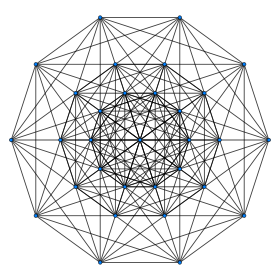Petrie polygon projection
Type Uniform 6-polytope
Family demihypercube
Schläfli symbol {3,33,1}
h{4,3,3,3,3}
s{2,2,2,2,2}
Coxeter-Dynkin diagramCoxeter symbol 131
5-faces 44 12 {31,2,1}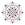32 {34}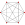4-faces 252 60 {31,1,1}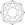192 {33}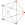Cells 640 160 {31,0,1}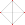480 {3,3}Faces 640 {3}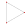Edges 240
Vertices 32
Vertex figure Rectified 5-simplex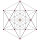Symmetry group D6, [35,1,1] = [1+,4,34]
+
Petrie polygon decagon
Properties convex

In geometry, a 6-demicube or demihexteract is a uniform 6-polytope, constructed from a 6-cube (hexeract) with alternate vertices deleted. It is part of a dimensionally infinite family of uniform polytopes called demihypercubes.

Coxeter named this polytope as 131 from its Coxeter-Dynkin diagram, with a ring on one of the 1-length Coxeter-Dynkin diagram branches. It can named similarly by a 3-dimensional exponential Schläfli symbol, {3,33,1}.

Cartesian coordinates

Cartesian coordinates for the vertices of a demihexeract centered at the origin are alternate halves of the hexeract:

(±1,±1,±1,±1,±1,±1)

with an odd number of plus signs.

Images

orthographic projections
Coxeter plane B6
Graph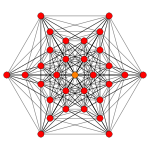Dihedral symmetry [12/2]
Coxeter plane D6 D5
Graph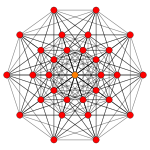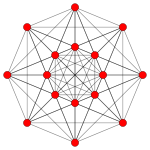Dihedral symmetry  
Coxeter plane D4 D3
Graph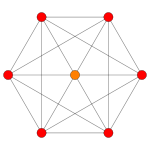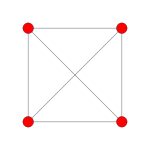Dihedral symmetry  
Coxeter plane A5 A3
Graph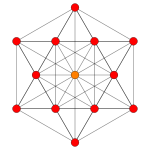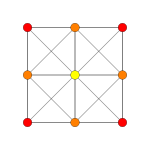Dihedral symmetry  

Related polytopes

There are 47 uniform polytopes with D6 symmetry, 31 are shared by the B6 symmetry, and 16 are unique:

Wikimedia Foundation. 2010.

Look at other dictionaries:

• 5-demicube — Demipenteract (5 demicube) Petrie polygon projection Type Uniform 5 polytope Family (Dn) 5 demicube Families (En) k21 polytope 1k2 poly …   Wikipedia

• 7-demicube — Demihepteract (7 demicube) Petrie polygon projection Type Uniform 7 polytope Family demihypercube Coxeter symbol 141 Schläfli symbol …   Wikipedia

• 9-demicube — Demienneract (9 demicube) Petrie polygon Type Uniform 9 polytope Family demihypercube Coxeter symbol 161 Schläfli symbol …   Wikipedia

• 8-demicube — Demiocteract (8 demicube) Petrie polygon projection Type Uniform 8 polytope Family demihypercube Coxeter symbol 151 …   Wikipedia

• 10-demicube — Demidekeract (10 demicube) Petrie polygon projection Type Uniform 10 polytope Family demihypercube Coxeter symbol 171 Schläfli symbol …   Wikipedia

• Demihypercube — Not to be confused with Hemicube (geometry). Alternation of the n cube yields one of two n demicubes, as in this 3 dimensional illustration of the two tetrahedra that arise as the 3 demicubes of the 3 cube. In geometry, demihypercubes (also… …   Wikipedia

• Coxeter group — In mathematics, a Coxeter group, named after H.S.M. Coxeter, is an abstract group that admits a formal description in terms of mirror symmetries. Indeed, the finite Coxeter groups are precisely the finite Euclidean reflection groups; the symmetry …   Wikipedia

• 16-cell — Regular hexadecachoron (16 cell) (4 orthoplex) Schlegel diagram (vertices and edges) Type Convex regular 4 polytope Schläfli symbo …   Wikipedia

• Cube — This article is about the geometric shape. For other uses, see Cube (disambiguation). Regular Hexahedron (Click here for rotating model) Type Platonic solid Elements F = 6, E = 12 V = 8 (χ = 2) …   Wikipedia

• Tetrahedron — For the academic journal, see Tetrahedron (journal). Regular Tetrahedron (Click here for rotating model) Type Platonic solid Elements F = 4, E = 6 V = 4 (χ = 2) Faces by s …   Wikipedia